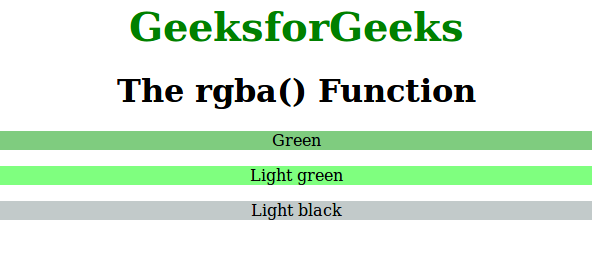Skip to content
Related Articles
CSS | rgba() Function
• Last Updated : 31 Mar, 2021

The rgba() function is an inbuilt function in CSS which is used to define the colors using the Red-Green-Blue-Alpha (RGBA) model.
Syntax:

`rgba( red, green, blue, alpha )`

Parameters: This function accepts four parameters as mentioned above and described below:

• red: This parameter is used to define the intensity of red color. Its is an integer value lies between 0 to 255, or as a percentage value between 0% to 100%.
• green: This parameter is used to define the intensity of green color. Its is an integer value lies between 0 to 255, or as a percentage value between 0% to 100%.
• blue: This parameter is used to define the intensity of blue color. Its is an integer value lies between 0 to 255, or as a percentage value between 0% to 100%.
• alpha: This parameter is used to defines the opacity and the value lies between 0.0 (fully transparent) to 1.0 (fully opaque).

Below program illustrates the rgba() function in CSS:
Program:

## html

 ```<``html``>``    ``<``head``>``        ``<``title``>rgba function``        ``<``style``>``            ``.gfg1 {``                ``background-color:rgba(1, 153, 0, 0.5);``                ``text-align:center;``            ``}``            ``.gfg2 {``                ``background-color:rgba(0, 255, 0, 0.5);``                ``text-align:center``            ``}``            ``.gfg3 {``                ``background-color:rgba(133, 150, 150, 0.5);``                ``text-align:center``            ``}``            ``.gfg {``                ``font-size:40px;``                ``font-weight:bold;``                ``color:green;``                ``text-align:center;``            ``}``            ``h1 {``                ``text-align:center;``            ``}``        ````    ````    ``<``body``>``        ``<``div` `class` `= ``"gfg"``>GeeksforGeeks``        ``<``h1``>The rgba() Function``        ``<``p` `class` `= ``"gfg1"``>Green` `        ``<``p` `class` `= ``"gfg2"``>Light green` `        ``<``p` `class` `= ``"gfg3"``>Light black` `    ````                   `

Output:Supported Browsers: The browser supported by rgba() function are listed below:

• Chrome 1.0 and above
• Internet Explorer 9.0 and above
• Firefox 3.0 and above
• Safari 3.1 and above
• Opera 10.0 and above

My Personal Notes arrow_drop_up